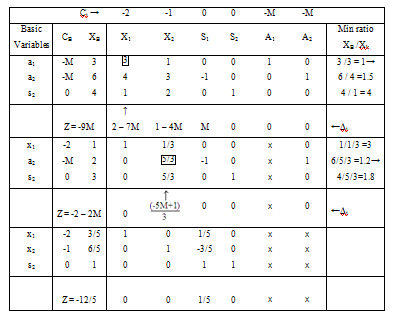#### Computational Procedure of Big M Method

Computational Procedure of Big - M Method, Charne's Penalty Method

Step 1 - State the problem in the standard form.

Step 2 - Then add non-negative artificial variable to the left side of every equations matching to the constraints of the type '≥' or '='.

While artificial variables are added, it produces violation of the corresponding constraints. This complexity is removed by bring in a condition which assures that artificial variables will be zero in the closing solution (provided the solution of the problem exists).

Alternatively, if the problem does not have any solution, then at least one of the artificial variables will appear in the end solution with positive value. This is attained by assigning a high price (per unit penalty) to these variables in the objective function. These large price will be designated through -M for maximization problems (+M for minimizing problem), where M > 0.

Step 3 - At last, use the artificial variables for the initial solution and continue with the usual simplex routine until the optimal solution is achieved.

Worked Examples

Example 1

Max Z = -2x1 - x2

Subject to

3x1 + x2 = 3

4x1 + 3x2 ≥ 6

x1 + 2x2 ≤ 4

&     x1 ≥ 0, x≥ 0

SLPP

Max Z = -2x1 - x2 + 0s1 + 0s2 - M a1 - M a2

Subject to

3x1 + x2 + a1= 3

4x1 + 3x2 - s1 + a2 = 6

x1 + 2x+ s2 = 4

x1 , x2 , s1, s2, a1, a≥ 0As it is known that, all Δj ≥ 0, optimal basic feasible solution is achieved

Thus the solution is Max Z = -12/5, x1 = 3/5, x2 = 6/5